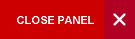Mathematics for Scientists and Engineers

Semester 1

MATH 1185 introduces students to engineering mathematics.  It reinforces the knowledge of complex numbers and differential equations gained from high school mathematics.  In addition, the student will be exposed to new methods from linear algebra, differential calculus of functions of one and several variables, systems of differential equations and their engineering applications.

Syllabus:
• Complex numbers; complex plane; exponential functions; Polar form, de Moivre’s formula; fractional powers of complex numbers; systems of linear equations; Gaussian elimination
• Vectors in 3-space (including vector equations of lines and planes); vectors in n-space; generalized vector spaces; span and subspaces; linear independence; bases and dimension
• Matrix Algebra; Transpose; Determinants; Rank and its application to non-homogeneous linear systems; Inverse Matrices; LU-factorization
• Basic differential calculus for functions of one and several variables
• Taylor series for functions of one and several variables; maxima and minima for functions of several variables
• Linear first ordinary differential equations; integrating factors; applications to electrical circuits; separable equations; linear ordinary differential equations of higher order; independence of solutions;
• Homogeneous Equations: general homogeneous equations; constant coefficient homogeneous ode; non-constant coefficient homogeneous ode; solution of non-homogeneous equations by undetermined coefficients; solution of homogeneous first order systems by using eigenvectors
Evaluation:

Final examination:  75%

Other Coursework:  25%

Learning Objectives:

Upon successful completion of MATH 1185, students will be able to:

• Solve linear first order differential equations using separation of variables
• Solve basic problems in differential calculus of functions of one and several variables.
• Comprehend the use and application of complex numbers to engineering problems.
• Comprehend the use and application of vectors to engineering problems.
• Comprehend the use and application of matrices to engineering problems.
CAPE/A-Level Maths or M08B/MATH0100 and M08C/MATH0110